# icwt

Inverse continuous 1-D wavelet transform

## Syntax

``xrec = icwt(wt)``
``xrec = icwt(wt,wname)``
``xrec = icwt(wt,f,freqrange)``
``xrec = icwt(wt,period,periodrange)``
``xrec = icwt(___,Name,Value)``

## Description

example

````xrec = icwt(wt)` inverts the continuous wavelet transform (CWT) coefficient matrix `wt` using default values. `icwt` assumes that you obtained the CWT using `cwt` with the default analytic Morse (3,60) wavelet. This wavelet has a symmetry of 3 and a time bandwidth of 60. `icwt` also assumes that the CWT uses default scales. If `wt` is a 2-D matrix, `icwt` assumes that the CWT was obtained from a real-valued signal. If `wt` is a 3-D matrix, `icwt` assumes that the CWT was obtained from a complex-valued signal. For a 3-D matrix, the first page of the `wt` is the CWT of the positive (counterclockwise) component and the second page of `wt` is the negative (clockwise) component. The pages represent the analytic and anti-analytic parts of the CWT, respectively.```

example

````xrec = icwt(wt,wname)` uses the analytic wavelet `wname` to invert the CWT. The specified wavelet must be the same as the wavelet used in `cwt`. Valid options for `wname` are `'morse'`, `'amor'`, and `'bump'`, which specify the Morse, Morlet, and bump wavelet, respectively.```

example

````xrec = icwt(wt,f,freqrange)` inverts the CWT over the frequency range specified in `freqrange`. `f` is the scale-to-frequency conversion obtained from `cwt`.```

example

````xrec = icwt(wt,period,periodrange)` inverts the CWT over the range of periods specified in `periodrange`. `period` is an array of durations obtained from `cwt` with a duration input. The `period` is the `cwt` output obtained using a `duration` input. The period range must be increasing and contained in `period`.```

example

````xrec = icwt(___,Name,Value)` returns the inverse CWT with additional options specified by one or more `Name,Value` pair arguments.```

## Examples

collapse all

Obtain the CWT of a speech sample and invert the CWT using the default analytic Morse wavelet.

```load mtlb; wt = cwt(mtlb); xrec = icwt(wt);```

Obtain the continuous wavelet transform of a speech sample and reconstruct the sample using the bump wavelet instead of the default Morse wavelet.

```load mtlb dt = 1/Fs; t = 0:dt:numel(mtlb)*dt-dt;```

Obtain the CWT.

`bumpmtlb = cwt(mtlb,Fs,'bump');`

Obtain the inverse CWT. Add the signal mean to the output.

`xrec = icwt(bumpmtlb,'bump','SignalMean',mean(mtlb));`

Plot the original and reconstructed signals.

```plot(t,mtlb) xlabel('Seconds') ylabel('Amplitude') hold on plot(t,xrec,'r') legend('Original','Reconstruction')```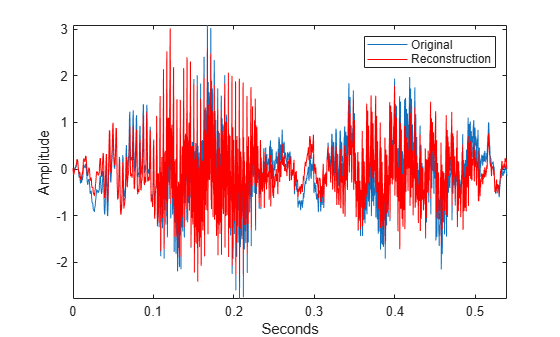Play and compare the original and reconstructed signals.

```p = audioplayer(mtlb,Fs); play(p) pause(2) px = audioplayer(xrec,Fs); play(px)```

Reconstruct a frequency-localized approximation to the Kobe earthquake data by extracting information from the CWT. The extracted information corresponds to frequencies in the range [0.030 0.070] Hz.

`load kobe;`

Obtain the CWT. Then, obtain the inverse CWT and add the signal mean back into the reconstructed data. The CWT does not preserve the signal mean.

```[wt,f] = cwt(kobe,1); xrec = icwt(wt,f,[0.030 0.070],'SignalMean',mean(kobe));```

Plot the original and reconstructed data.

```subplot(211) plot(kobe); grid on title('Original Data'); ylabel('Amplitude') subplot(212) plot(xrec); grid on title('Bandpass Filtered Reconstruction [0.030 0.070] Hz'); xlabel('Frequency'); ylabel('Amplitude');```Use the inverse continuous wavelet transform to reconstruct an approximation to El Nino data based on 2 to 8 year periods.

Load the El Nino data and obtain its CWT. The data is sampled monthly. To obtain the periods in years, specify the sampling interval as 1/12 of a year.

```load ninoairdata; [cfs,period] = cwt(nino,years(1/12));```

Obtain the inverse CWT for periods of 2 to 8 years.

`xrec = icwt(cfs,period,[years(2) years(8)]);`

Plot the CWT of the reconstructed data and compare it to the CWT of the original data.

`cwt(nino,years(1/12)); title('Original Data');`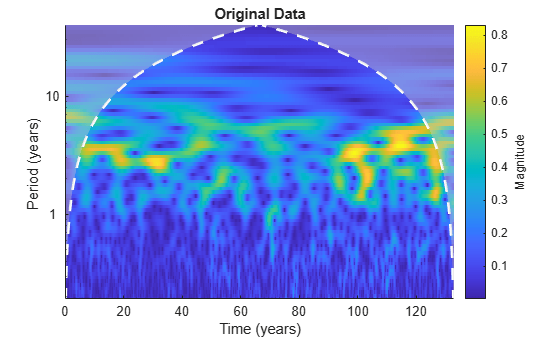```figure; cwt(xrec,years(1/12)); title('Approximation Based on 2-8 Year Periods');```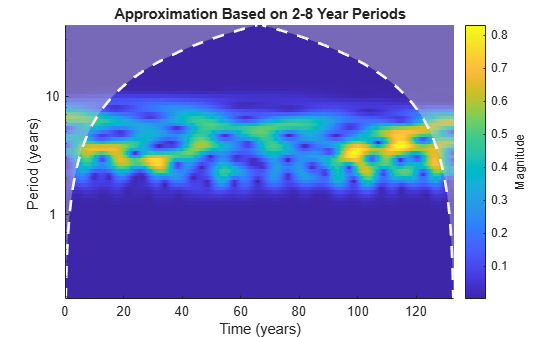Compare the original data with the reconstructed data in time.

```figure; subplot(211) plot(datayear,nino); grid on; ax = gca; ax.XTickLabel = ''; axis tight; title('Original Data'); subplot(212) plot(datayear,xrec); grid on; axis tight; xlabel('Year'); title('El Nino Data - 2-8 Year Periods');```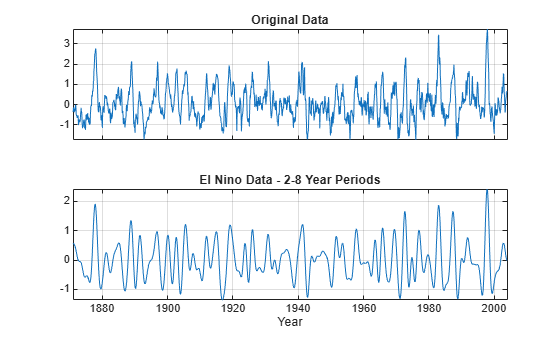Add a trend to the continuous wavelet transform of a complex-valued dataset and reconstruct.

Obtain the CWT of the NPG2006 dataset.

```load npg2006.mat wt = cwt(npg2006.cx);```

Create a time-varying trend derived from the data.

`trend = smoothdata(npg2006.cx,'movmean',100);`

Obtain the inverse CWT and add the trend. Plot the original data and the reconstructed data.

```xrec = icwt(wt,'SignalMean',trend); plot([real(xrec)' real(npg2006.cx)]) grid on legend('Trend','Original')```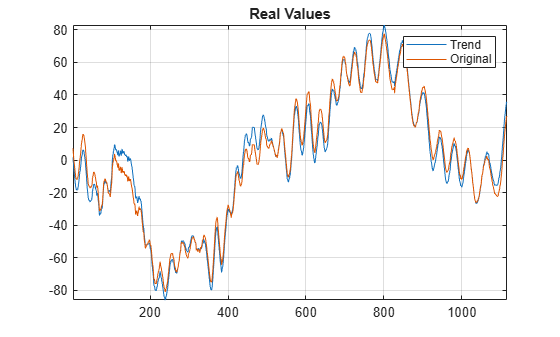```figure plot([imag(xrec)' imag(npg2006.cx)]) grid on legend('Trend','Original')```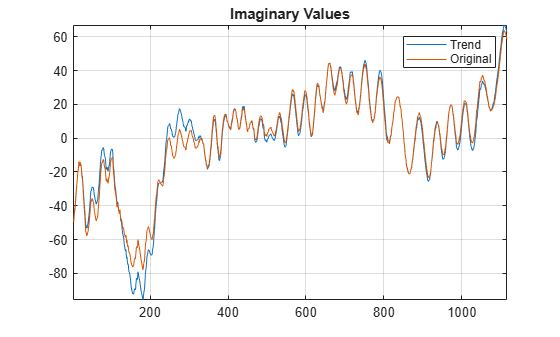## Input Arguments

collapse all

Continuous wavelet transform coefficients, specified as a matrix of complex values. `wt` is the output from the `cwt` function.

Data Types: `double`
Complex Number Support: Yes

Analytic wavelet used to invert the CWT, specified as `'morse'`, `'amor'`, or `'bump'`. These character vectors specify the analytic Morse, Morlet, and bump wavelet, respectively. The specified wavelet must be the same type of wavelet used to obtain the `cwt`.

The default Morse wavelet uses a default symmetry parameter, $\gamma$, that is 3 and has a default time bandwidth of 60.

CWT frequencies, specified as a vector. The number of elements in the frequency vector must equal to the number of rows in the input CWT coefficient matrix, `wt`. If you specify `f`, you must also specify `freqrange`.

Frequency range for which to return inverse continuous wavelet transform values, specified as a two-element vector or 2-by-2 matrix. If `wt` is a 2-D matrix, `freqrange` must be a two-element vector. If `wt` is a 3-D matrix, `freqrange` can be a two-element vector or a 2-by-2 matrix. If `wt` is a 3-D matrix and `freqrange` is a vector, inversion is performed over the same frequency range in both the positive (analytic) and negative (anti-analytic) components of `wt`. If `freqrange` is a 2-by-2 matrix, the first row contains the frequency range for the positive part of `wt` (first page) and the second row contains the frequency range for the negative part of `wt` (second page). For a vector, the elements of `freqrange` must be strictly increasing and contained in the range of the frequency vector `f`. For a matrix, each row of `freqrange` must be strictly increasing and contained in the range of `f`. `f` is the scale-to-frequency conversion obtained in CWT. For the inversion of a complex-valued signal, you can specify one row of `freqrange` as a vector of zeros. If the first row of `freqrange` is a vector of zeros, only the negative (anti-analytic part) is used in the inversion. If you specify `freqrange`, you must also specify `f`.

For example `[0 0; 1/10 1/4]` inverts the negative (clockwise) component over the frequency range``` [1/10 1/4]```. The positive (counterclockwise) component is first set to all zeros before performing the inversion. Similarly, ```[1/10 1/4; 0 0]``` inverts the CWT by selecting the frequency range `[1/10 1/4]` from the positive (counterclockwise) component and setting the negative component to all zeros.

Time periods corresponding to the rows of CWT coefficient matrix `wt`, specified as a vector. `period` is the output of `cwt`, when the CWT is obtained using a `duration` input.

Period range for which to return inverse continuous wavelet transform values, specified as a two-element vector or 2-by-2 matrix. If `wt` is a 2-D matrix, `periodrange` must be a two-element vector of durations. If `wt` is a 3-D matrix, `periodrange` can be a two-element vector of durations or 2-by-2 matrix of durations. If `periodrange` is a vector of durations and `wt` is a 3-Dmatrix, inversion is performed over the same frequency range in both the positive (analytic) and negative (anti-analytic) components of `wt`. If `periodrange` is a 2-by-2 matrix of durations, the first row contains the period range for the positive part of `wt` (first page) and the second row contains the period range for the negative part of `wt` (second page). For a vector, the elements of `periodrange` must be strictly increasing and contained in the range of the period vector `period`. The elements of `periodrange` and `period` must have the same units. For a matrix, each row of `periodrange` must be strictly increasing and contained in the range of the period vector P. For the inversion of a complex-valued signal, you can specify one row of `periodrange` as a vector of zero durations. If the first row of `periodrange` is a vector of zero durations, only the negative (anti-analytic part) is used in the inversion. If you specify `periodrange`, you must also specify `period`.

For example ```[seconds(0) seconds(0); seconds(1/10) seconds(1/4)]``` inverts the negative(clockwise) component over the period range `[seconds(1/10) seconds(1/4)]`. The positive (counterclockwise) component is first set to all zeros before performing the inversion. Similarly, ```[seconds(1/10) seconds(1/4); seconds(0) seconds(0)]``` inverts the CWT by selecting the period range `[1/10 1/4]` from the positive (counterclockwise) component and setting the negative component to all zeros.

### Name-Value Pair Arguments

Specify optional comma-separated pairs of `Name,Value` arguments. `Name` is the argument name and `Value` is the corresponding value. `Name` must appear inside quotes. You can specify several name and value pair arguments in any order as `Name1,Value1,...,NameN,ValueN`.

Example: `'TimeBandwidth',45` sets the time bandwidth to 45.

Time bandwidth of the Morse wavelet, specified as a comma-separated pair consisting of `'TimeBandwidth'` and a scalar greater than 3 and less than or equal to 120. The specified time bandwidth must be the same as the time bandwidth used in the `cwt`. The symmetry of the Morse wavelet is assumed to be 3.

You cannot specify both the `'TimeBandwidth'` and `'WaveletParameters'`. If you specify `'TimeBandwidth'` , you cannot specify `'WaveletParameters'`. To specify both the symmetry and time bandwidth, use `'WaveletParameters'` instead.

Symmetry and time bandwidth of Morse wavelet, specified as the comma-separated pair consisting of `'WaveletParameters'` and a two-element vector of scalars. The first element of the vector is the symmetry, $\gamma$, and the second element is the time-bandwidth. The specified wavelet parameters must be the same as the parameters used in `cwt`.

You cannot specify both `'WaveletParameters'` and `'TimeBandwidth'`. If you specify `'WaveletParameters'`, you cannot specify `'TimeBandwidth'`. To specify the time bandwidth and use the default symmetry value of 3, use `'TimeBandwidth'` instead.

Signal mean to add to the `icwt` output, specified as the comma-separated pair consisting of `'SignalMean'` and a scalar or vector. If signal mean is a vector, it must be the same length as the column size of the wavelet coefficient matrix. If `wt` is a 2-D matrix, the signal mean must be a real-valued scalar or vector. If `wt` is a 3-D matrix, the signal mean must be a complex-valued scalar or vector. Because `cwt` does not preserve the signal mean, `icwt` does not contain the signal mean by default. Adding a non-zero signal mean to a frequency- or period-limited reconstruction adds a zero-frequency component to the reconstruction.

Scaling coefficients to use in the inverse CWT, specified as a real- or complex-valued vector, obtained as an optional output of `cwt`.

The scaling coefficient output is only supported for Morse wavelets and the analytic Morlet wavelet. The length of `ScalingCoefficients` is equal to the column size of `wt`.

You cannot specify both the `'SignalMean'` and `'ScalingCoefficients'` name-value pairs.

Number of voices per octave, specified as the comma-separated pair consisting of `'VoicesPerOctave'` and an even integer from 4 to 48. The CWT scales are discretized using the specified number of voices per octave. The number of voices per octave must be the same as the number of voices per octave used to obtain the CWT.

You cannot specify the number of voices per octave if you specify either the frequency, `f`, or duration, `period`.

## Output Arguments

collapse all

Inverse 1-D continuous wavelet transform, returned as a real- or complex-valued row vector.

Data Types: `double`

collapse all

### Inverse Continuous Wavelet Transform

`icwt` computes the inverse CWT based on a discretized version of the single integral formula due to Morlet . For a brief description of the theoretical foundation for the single integral formula, see Inverse Continuous Wavelet Transform. For additional theoretical information, see section 2.4 of . The discretized version of this integral is presented in .

 Lilly, J. M., and S. C. Olhede. "Generalized Morse Wavelets as a Superfamily of Analytic Wavelets." IEEE Transactions on Signal Processing 60, no. 11 (November 2012): 6036–41. https://doi.org/10.1109/TSP.2012.2210890.

 Lilly, J.M., and S.C. Olhede. "Higher-Order Properties of Analytic Wavelets." IEEE Transactions on Signal Processing 57, no. 1 (January 2009): 146–60. https://doi.org/10.1109/TSP.2008.2007607.

 Lilly, J. M. jLab: A data analysis package for Matlab, version 1.6.2. 2016. http://www.jmlilly.net/jmlsoft.html.

 Lilly, J. M., and J.-C. Gascard. "Wavelet Ridge Diagnosis of Time-Varying Elliptical Signals with Application to an Oceanic Eddy." Nonlinear Processes in Geophysics 13, no. 5 (September 14, 2006): 467–83. https://doi.org/10.5194/npg-13-467-2006.

 Duval-Destin, M., M. A. Muschietti, and B. Torresani. “Continuous Wavelet Decompositions, Multiresolution, and Contrast Analysis.” SIAM Journal on Mathematical Analysis 24, no. 3 (May 1993): 739–55. https://doi.org/10.1137/0524045.

 Daubechies, Ingrid. Ten Lectures on Wavelets. CBMS-NSF Regional Conference Series in Applied Mathematics 61. Philadelphia, Pa: Society for Industrial and Applied Mathematics, 1992.

 Torrence, Christopher, and Gilbert P. Compo. “A Practical Guide to Wavelet Analysis.” Bulletin of the American Meteorological Society 79, no. 1 (January 1, 1998): 61–78. https://doi.org/10.1175/1520-0477(1998)079<0061:APGTWA>2.0.CO;2.

 Holschneider, M., and Ph. Tchamitchian. “Pointwise Analysis of Riemann’s 'Nondifferentiable' Function.” Inventiones Mathematicae 105, no. 1 (December 1991): 157–75. https://doi.org/10.1007/BF01232261.

## SupportGet trial now